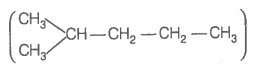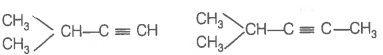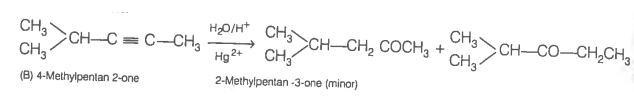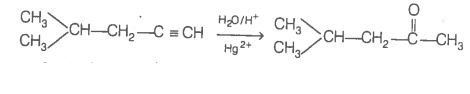896 mL vapour of a hydrocarbon 'A' having carbon 87.80% and hydrogen 12.19% weighs 3.28g at STP. Hydrogenation of 'A' gives 2-methylpentant. Also 'A' on hydration in the presence of ${\mathrm{H}}_{2}{\mathrm{SO}}_{4}$ and ${\mathrm{HgSO}}_{4}$ gives a ketone 'B' having molecular formula ${\mathrm{C}}_{6}{\mathrm{H}}_{12}\mathrm{O}$. The ketone 'B' gives a positive iodoform test. Find the structure of 'A' and give the reactions involved.

To determine the molecular mass of hydrogen (A) 896 mL vapour of ${\mathrm{C}}_{\mathrm{x}}{\mathrm{H}}_{\mathrm{y}}\left(\mathrm{A}\right)$ weighs 3.28 g at STP
22700 mL vapour of ${\mathrm{C}}_{\mathrm{x}}{\mathrm{H}}_{\mathrm{y}}\left(\mathrm{A}\right)$ weighs  at STP
Hence, molecular mass of . To determine the empirical formula of hydrocarbon (A).
 Element % Atomic mass Relative ratio Relative no. of atoms Simplest ratio C 87.8 12 7.31 1 3 H 12.18 1 12.19 1.66 4.98$\approx$5

Thus, Empirical formula of A is ${\mathrm{C}}_{3}{\mathrm{H}}_{5}$.

$\therefore$ Empirical formula mass$=36+5=41.$

Molecular mass is double of empirical formula mass.

$\therefore$ Molecular formula is ${\mathrm{C}}_{6}{\mathrm{H}}_{10}$

To determine the structure of compounds (A) and (B)Hence, hydrogenation of hydrocarbon (A) requires 2 moles of hydrogen to form 2-methylpentane. Therefore, hydrocarbon (A) is an alkyne having five carbon atoms in a straight chain and a methyl substituent at position 2. Thus, the possible structures for the alkyne (A) are I and II.Since, addition of H2O to alkyne (A) in presence of Hg2+, give a ketone which gives positive iodoform test, therefore, ketone (B) must be a methyl ketone, i.e., it must contain a COCH3 group.

Now addition of H2O to alkyne (II) should give a mixture of two ketones in which 2-methylpentan-3 one (minor) and 4-methylpentan-2-one ketone (B) (which shows +ve iodoform test) predominates.In contrast, addition of H2O to alkyne (I) will give only one ketone, i.e., 4-methylpentan-2-one which gives iodoform test.Thus, hydrocarbon ${\mathrm{C}}_{\mathrm{x}}{\mathrm{H}}_{\mathrm{y}}\left(\mathrm{A}\right)$ is 4-methylpent-1-yne. 4-methylpentan-2 one (gives +ve iodoform test)